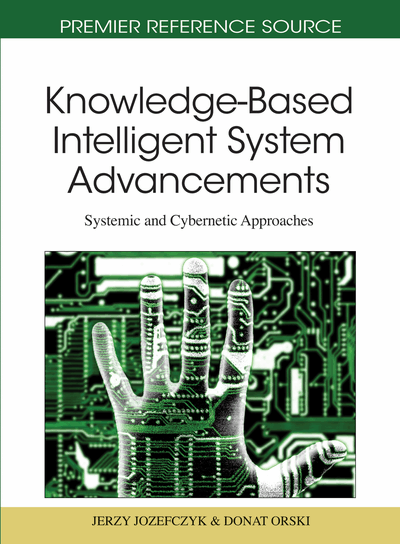# Active Learning in Discrete-Time Stochastic Systems

Tadeusz Banek (Lublin University of Technology, Poland) and Edward Kozlowski (Lublin University of Technology, Poland)
DOI: 10.4018/978-1-61692-811-7.ch016
Available
\$29.50
No Current Special Offers

Chapter Preview
Top

## Introduction

Learning is widely recognized as an important issue in modern, knowledge based societies. There is extensive literature on this subject in the areas of Management Sciences, System Sciences, Cybernetics, widely describing a need for investigations of learning processes. It is conjectured that the quality, speed and universality of these processes are crucial factors for comparison of modern and past societies.

Here is the right moment for reflection. If the self-learning processes are important and worth understanding for modern, knowledge based societies, but to difficult for studying directly, why do not try to understand them lean on the examples solved in Adaptive Control Theory? The following questions appear immediately; can these processes be described or investigated quantitatively? What means “passive” or “active” learning? There is any hope to apply the mathematical techniques helping to understand the essence of learning?

The aim of this chapter is to convince the reader that the answer could be positive and to propose an approach which is based on the ideas of adaptive control theory. To make the problem of active learning more specific we state a stochastic control problem with unknown parameters. This means we consider controlled systems having parameters which are unknown to the controller. They are modeled as random variables with known distribution functions (a’priori). The control law which has to optimize some objective function must take into account all available information, including information (posteriori) about parameters. More precise information about parameters, better results of control actions measured by the objective function. Observing system's trajectory the controller improve his knowledge about the parameters. Selecting trajectories he can choose the best one. But how to learn on line (!) the values of unknown parameters and how to do it in the most efficient way? These are the fundamental questions in the adaptive control theory. We believe in importance of this question and its universality on the general level - independently on any connections with optimal control (adaptive or not). Moreover, we hope that understanding this problem can - and must - help in much more complex and advanced problems of learning in knowledge based societies.

The chapter is organized as follows. In section 2 we state the adaptive control problem for nonlinear systems which are affine with respect to controls and disturbances. Applying weak variations, a technique from Calculus of Variations, we obtain a necessary condition of optimality. Following a seminal paper by Rishel (1986), we transfer this condition into an algorithm for computing extremal controls in section 3. In sections 4 and 5 a concept of incremental value of information is introduced. Roughly speaking, it is an approximate amount of money one has to pay for the exact knowledge of the parameters value. This value can be used for several purposes, for instance, a comparison of the net profit with the extra cost of possible purchasing of this information. In the next section we apply our general results to a simple one dimensional LQG problem. This shows several surprising effects of imperfect information and consequences of learning. For instance, the certainty equivalence principle, which was widely recognized methodological candidate for finding an optimal adaptive control is not valid in this case. Numerical simulations based on Rishel's algorithm suggest an alternative candidate that is explained in Conclusions. Finally, in the last section we introduce the so-called self-learning. This is done by considering the control problems with the conditional entropy, entering explicitly in the performance criteria. In this manner the self-learning, being the auxiliary objective, associated with the main objective in the task considered in classical automatics, became here the objective unto itself, the fundamental objective. The resulting trajectories say a lot about ξ, but, in contrast to the case analyzed in our previous paper Banek & Kozłowski (2005), where the joint entropy minimization problem was considered, nowcan be arbitrarily large. For non-technical systems (economical, social, etc.) such a formulation of the self-learning problem is natural. We show that this problem and its generalization can be treated as an optimal adaptive control problem, and solved by using Rishel's methodology (see e.g. Rishel, 1986; Harris & Rishel, 1986). Next, we present some results about modeling with conditional entropy and determining the optimal control for learning process without costs.

## Complete Chapter List

Search this Book:
Reset